# How to count cells with text and characters in Excel

This tutorial shows how to count cells with text and characters in Excel 2010-2013. You will find helpful Excel formulas for counting characters in one or several cells, character limits for cells and get a link to see how to find the number of cells that contain specific text.

Initially Excel was designed to work with numbers, thus you can always choose one of three ways to perform any counting or summing operation with digits. Fortunately, the developers of this helpful application didn't forget about text. Thus, I'm writing this article to show you how to use different options and formulas in Excel to count cells with text or count certain characters in a string.

Below you can find the options I'm going to cover:

At the end, you'll also find links to our previous blog posts related to counting cells in Excel.

### Excel - Count cells with text

If you need to count the number of cells with text in Excel, you can use the COUNTIF function:

`=COUNTIF(A2:D10,"*")`

Here A2:D10 is your range with data and "*" is a wildcard matching any number of characters.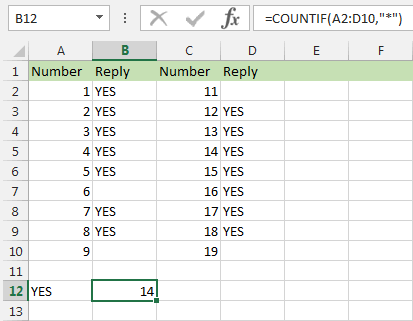Just enter the formula with the correct range to any cell where you want to see the result and press Enter. The result will be right there.

Note. The logical values TRUE and FALSE are not counted as text. In addition, numbers are not counted by "*" if they are not entered as text starting with an apostrophe ('). Empty cells beginning with an apostrophe (') will be counted.

One more formula you can use to count cells with text in Excel is

=COUNTA(range)-COUNT(range)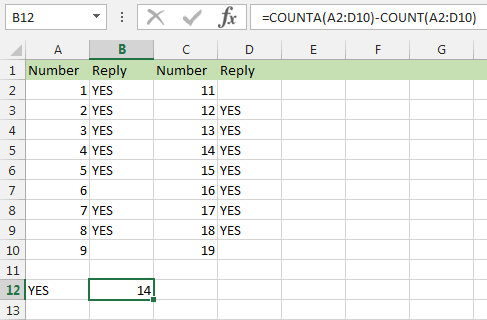This formula will ignore both dates and numbers.

Another function you can use in Excel to count the number of cells with text is an array formula that needs to be entered with Ctrl+Shift+Enter. You can use SUMPRODUCT to count text values along with the function ISTEXT like this:

=SUMPRODUCT(--ISTEXT(range))

The double hyphen, or double unary, coerces the result of ISTEXT from a logical value of TRUE or FALSE, to 1's and 0's. SUMPRODUCT then adds these values together to get a result.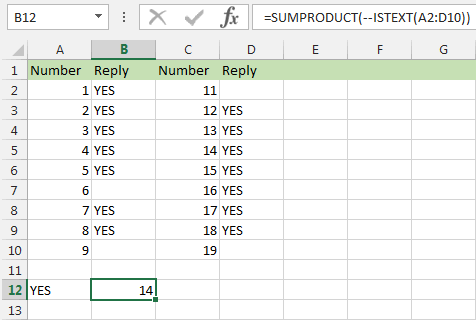Thus if you need to quickly count cells with text in Excel, feel free to use one of the formulas above.

### Excel formula to count characters in a cell

I can presume that in one of the future versions of Excel the Status Bar will show the number characters in a string. While we are hoping and waiting for the feature, you can use the following simple formula:

`=LEN(A1)`

In this formula A1 is the cell where the number of text characters will be calculated.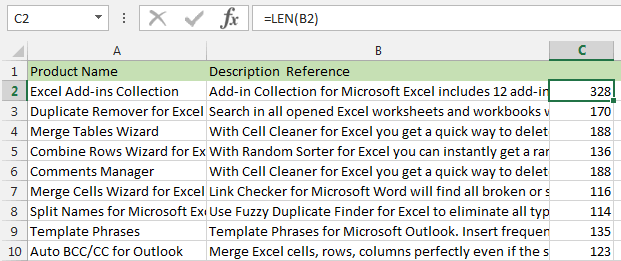The point is Excel has character limitations. For example, the header cannot exceed 254 characters. If you exceed the maximum, the header will be cut. The formula can be helpful when you have really long strings in your cells and need to make sure that your cells don't exceed 254 characters to avoid problems with importing or displaying your table in other sources.

Thus, after applying the function `=LEN(A1)` to my table, I can easily see the descriptions that are too long and need to be shortened. Thus, feel free to use this formula in Excel each time you need to count the number of characters in a string. Just create the Helper column, enter the formula to the corresponding cell and copy it across your range to get the result for each cell in your column.

### Excel - Count characters in several cells

You may also need to count the number of characters from several cells. In this case you can use the following formula:

=SUM(LEN(range))

Note. The above formula must be entered as an array formula. To enter it as an array formula, press Ctrl+Shift+Enter.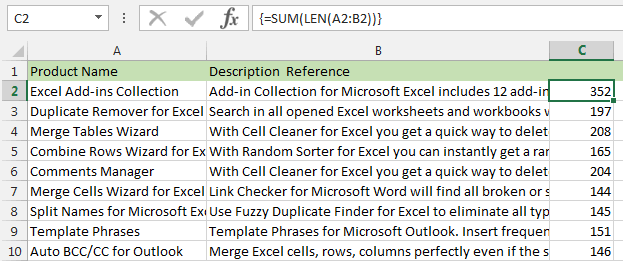This formula can be helpful if you want to see if any rows exceed the limitations before merging or importing your data tables. Just enter it to the Helper column and copy across using the fill handle.

### Excel formula to count certain characters in a cell

In this part, I'll show you how to calculate the number of times a single character occurs in a cell in Excel. This function really helped me when I got a table with multiple IDs that couldn't contain more than one zero. Thus, my task was to see the cells where zeros occurred and where there were several zeros.

If you need to get the number of occurrences of certain character in a cell or if you want to see if your cells contain invalid characters, use the following formula to count the number of occurrences of a single character in a range:

`=LEN(A1)-LEN(SUBSTITUTE(A1,"a",""))`

Here "a" is a character you need to count in Excel.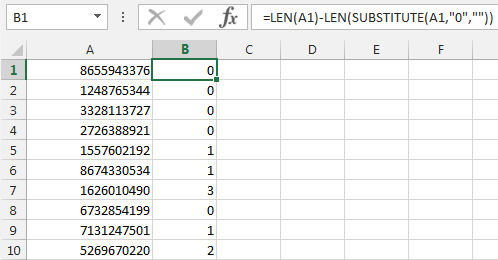What I really like about this formula is that it can count the occurrences of a single character as well as part of some text string.

### Count the number of occurrences of certain character in a range

If you want to count the number of occurrences of certain character in several cells or in one column, you can create a Helper column and paste there the formula I described in the previous part of the article `=LEN(A1)-LEN(SUBSTITUTE(A1,"a",""))`. Then you can copy it across the column, sum this column and get the expected result. Sounds too time consuming, doesn't it?

Fortunately, Excel often gives us more than one way to get the same result and there is a more simple option. You can count the number of certain characters in a range using this array formula in Excel:

=SUM(LEN(range)-LEN(SUBSTITUTE(range,"a","")))

Note. The above formula must be entered as an array formula. Please make sure you press Ctrl+Shift+Enter to paste it.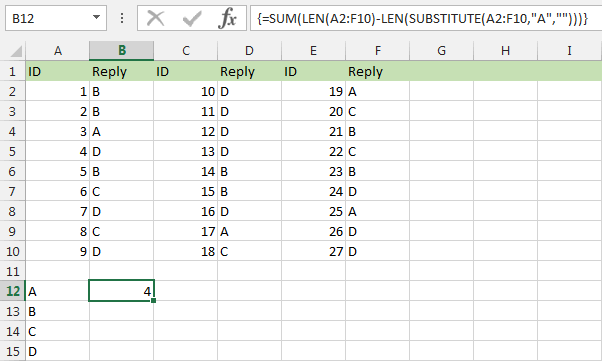### Count the number of occurrences of certain text in a range

The following array formula (must be entered with Ctrl+Shift+Enter) will help you count the number of occurrences of certain text in a range:

`=SUM((LEN(C2:D66)-LEN(SUBSTITUTE(C2:D66,"Excel","")))/LEN("Excel"))`

For example, you can count the number of times the word "Excel" is entered in your table. Please don't forget about space or the function will count words beginning with certain text, not the isolated words.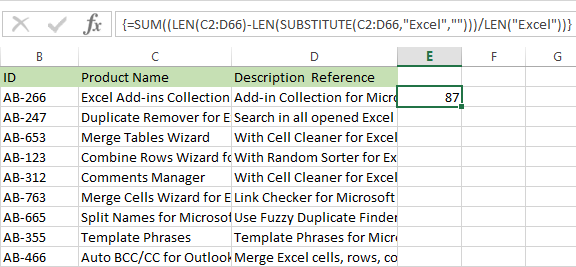Thus, if you have certain text snippet scattered around your table and need to count its occurrences really quickly, use the formula above.

### Excel character limits for cells

If you have worksheets with large amount of text in several cells, you may find the following information helpful. The point is that Excel has a limitation on the number of characters you can enter to a cell.

• Thus, the total number of characters that a cell can contain is 32,767.
• A cell can display only 1,024 characters. At the same time, the Formula bar can show you all 32,767 symbols.
• The maximum length of formula contents is 1,014 for Excel 2003. Excel 2007-2013 can contain 8,192 characters.

Please consider the facts above when you have long headers or when you are going to merge or import your data.

### Count cells that contain specific text

If you need to count the number of cells that contain certain text, feel free to use the COUNTIF function. You will find it beautifully described in COUNTIF formulas with wildcard characters (partial match).

Hope this article will help you next time you need to count the number of cells with text or certain character occurrences in your spreadsheet. I tried to cover all options that can help you - I described how to count cells with text, showed you an Excel formula for counting characters in one cell or in a range of cells, you found how to count the number of occurrences of certain characters in a range. Also you can benefit from one of the links to our previous posts to find many additional details.

That's all for today. Be happy and excel in Excel!